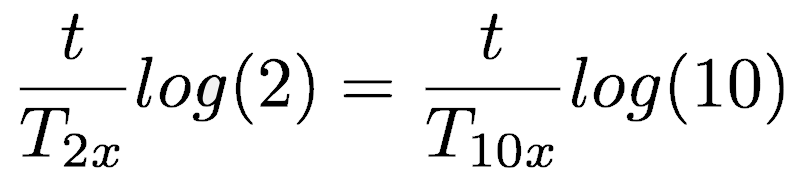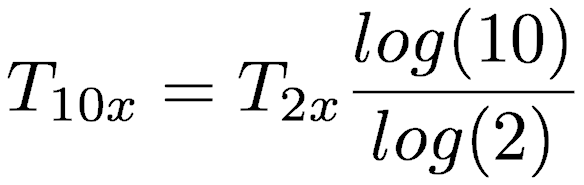# stratigrafia

DOUBLING TIME TO 10X TIME

3/26/20

Many of the recent articles on COVID-19 talk about the doubling time of the number of cases, but I wanted to think about it in terms of order of magnitude. The conversion is straightforward.

The starting point describes the number of cases at some time, N(t), by the number of cases at a starting time, N0, and the time it takes for the number of cases to double, T2xThe same equation could be written for the number of cases at some future time when given the time for the number of cases to increase by a factor of 10, T10xSetting these two equal givesCancelling the starting number of cases simplifies it toTaking the logarithm (any base) of both sidesRemoving t from both sides and solving for T10x gives what we are afterThis could be generalized between any two scales, such as the tripling time and quadrupling time, if that’s your thing, man.

Home